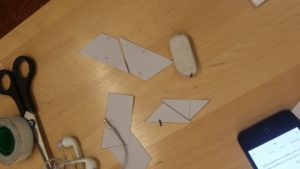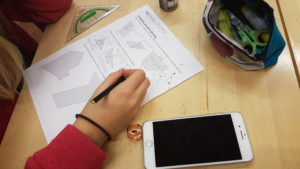Mathematics has always been seen as a theoretical subject. This is probably the biggest reason why a teacher always explaines new theory before students start practicing. To me and many others, mathematics is a practical subject. It is possible to discover rules, patterns and formulas without anyone telling you how it works. Making new discoveries on your own is of major importance in gaining confidence, interest and develop skills needed for high level research. This is why in the student-centered method you should try to present problems that guides your students forward and give them enough time to research and perhaps with a little guidence figure things out on their own.

Since we have a long tradition in using the behavioristic model, the constuctivistic view might feel strange for many teachers at first, and that is why I would like to present a sequence of math lessons where my students examine the area of different polygones by using some models and me guiding them forward, to give you an idea of what student-centered teaching can look like.

### The height of a polygonIn order to understand the area formulas you must first know what the polygones height is. The height is incredibly simple. Just place a polygon on the ground and measure the distance from the ground to the highest point of the polygon. The only thing you have to realize is that the ruler must be placed in a right angle from the ground. With this said students are ready to work. I have given them models and their job is to measure all of the heights. As you can probably understand this is all very easy until you get to the obtuse triangle since two of its heights will be on the outside. This is however much easier to figure out and understand if you have models to work with.Only after the students have developed an understanding for the content, is it time for the theoretical part. The next task is to write a correct definition of the height and use it to draw the heights, epecially on the obtuse triangle which we know can be difficult at first. For homework students will draw arbitrary triangles of all types and draw their heights and then we are ready to move on.

### The area of polygonesThe students already know the area formula for the rectangle so by using their previous knowledge it is possible to discover the area formula for a parallelogram, triangle and a trapezoid. The students are given a few models to work with and they are ready to work. After they have come to the conclusion that a parallelogram can be made into a rectangle, and two equal triangles or two equal trapezoids form a parallelogram they are now able to figure out the area formulas.Finally students will measure the distances needed to calculate the area of different shapes using their newly discovered formulas. After all of this students will have developed a strong knowledge of the area of polygones and are ready for more advanced problem solving and since they have developed a deep understanding of the content they will have an interesting time using their new knowledge in solving more complex problems.

Teaching according to the student-centered model is not to explain how mathematics works, but instead come up with problems that activates students interest and eager to learn.

Are you looking for material in maths designed according to the student-centered model? If you are, please visit Ma.fi (available in English, Swedish and Finnish) and learn about a digital math book for junior-high.

Student-centered teaching in practice